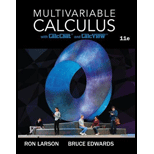# Wronskian The Wronskian of two differentiable functions f and g , denoted by W (f, g), is defined as the function given W ( f , g ) = | f g f ' g ' | . The function f and g are linearly independent when there exists at least one value of x for which W ( f , g ) ≠ 0 . In exercise 71-74, use the Wronskian to verify that the two functions are linearly independent. y 1 = e a x sin b x y 2 = e a x cos b x### Multivariable Calculus

11th Edition
Ron Larson + 1 other
Publisher: Cengage Learning
ISBN: 9781337275378

#### Solutions

Chapter
Section### Multivariable Calculus

11th Edition
Ron Larson + 1 other
Publisher: Cengage Learning
ISBN: 9781337275378
Chapter 16.2, Problem 73E
Textbook Problem
1 views

## Wronskian The Wronskian of two differentiable functions f and g, denoted by W (f, g), is defined as the function given W ( f , g ) = | f g f ' g ' | .The function f and g are linearly independent when there exists at least one value of x for which W ( f , g ) ≠ 0 . In exercise 71-74, use the Wronskian to verify that the two functions are linearly independent. y 1 = e a x sin b x y 2 = e a x cos b x

To determine

To verify:

The two function y1=eaxsinbxandy2=eaxcosbx are linearly independent by using Wronskian Method.

### Explanation of Solution

Given information:

The given function are y1=eaxsinbxandy2=eaxcosbx.

Concept Used:

The Wronskian of two differentiable functions f and g, denoted by W (f, g), is defined as the function given by the determinant W(f,g)=|fgf'g'|.

Proof:

First, find the derivative of the y1andy2 with respect to x, we get

y1'=beaxcosbx+aeaxsinbxandy2'=aeaxcosbxbeaxsinbx

Now, we have to find the determinant W(y1,y2)=|y1y2y1'y2'|.

W(y1,y2)=|eaxsinbxeaxcosbxbeaxcosbx+aeaxsinbx

### Still sussing out bartleby?

Check out a sample textbook solution.

See a sample solution

#### The Solution to Your Study Problems

Bartleby provides explanations to thousands of textbook problems written by our experts, many with advanced degrees!

Get Started

Find more solutions based on key concepts
In Exercises 75-98, perform the indicated operations and/or simplify each expression. 84. 89x2+23x+16xx2163x2x+...

Applied Calculus for the Managerial, Life, and Social Sciences: A Brief Approach

Suppose . Then F(x)g(x) + C f′(g(x)) + C F(g′(x)) + C F(g(x)) + C

Study Guide for Stewart's Single Variable Calculus: Early Transcendentals, 8th

Evaluate the integral. 5. x21x4dx

Single Variable Calculus: Early Transcendentals

Practice Solve each logarithmic equation. log3log3x=1

College Algebra (MindTap Course List)

In Exercises 1-22, evaluate the given expression. C(6,5)

Finite Mathematics for the Managerial, Life, and Social Sciences

The notation {xP(x)} is read _______

Discrete Mathematics With Applications

Reminder Round all answers to two decimal places unless otherwise indicated. Sales Growth A study of the sales ...

Functions and Change: A Modeling Approach to College Algebra (MindTap Course List)

Extending Model for Repair Time. This problem is an extension of the situation described in exercise 35. Develo...

Modern Business Statistics with Microsoft Office Excel (with XLSTAT Education Edition Printed Access Card) (MindTap Course List)

In Problems 18 state the order of the given ordinary differential equation. Determine whether the equation is l...

A First Course in Differential Equations with Modeling Applications (MindTap Course List)